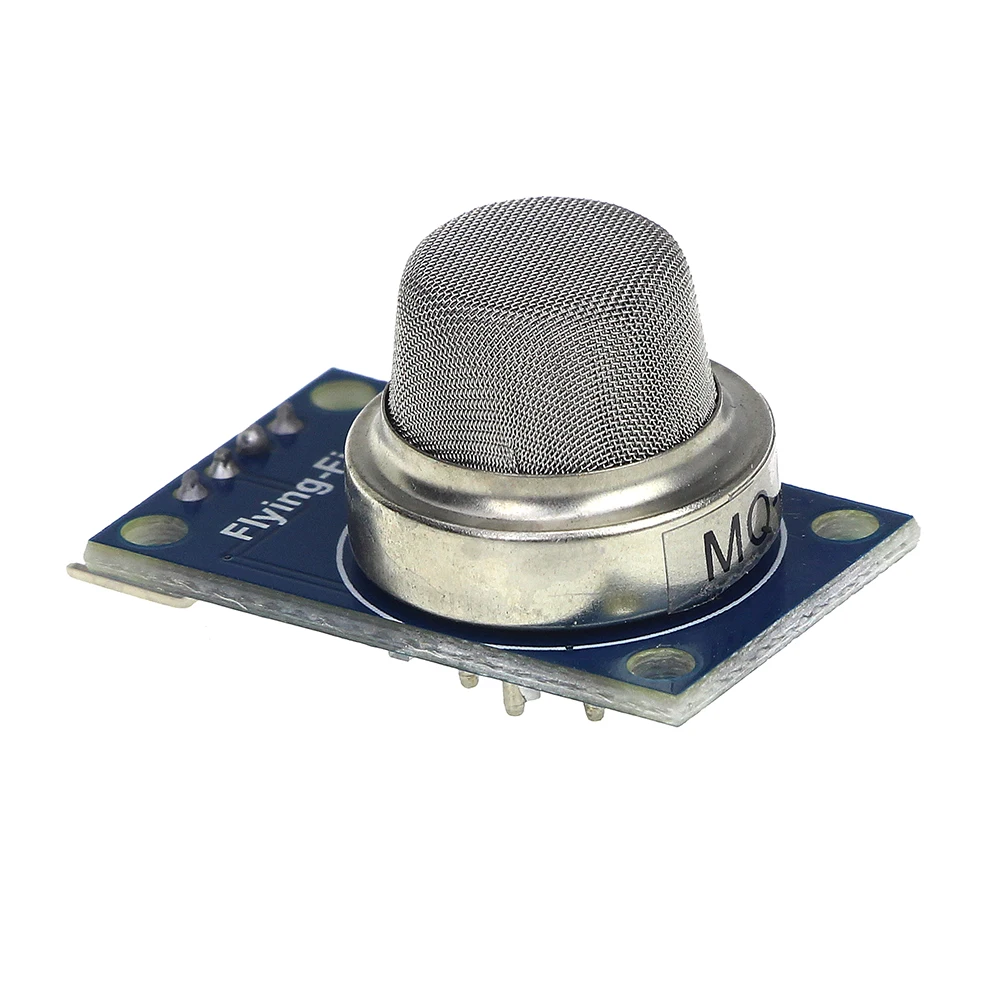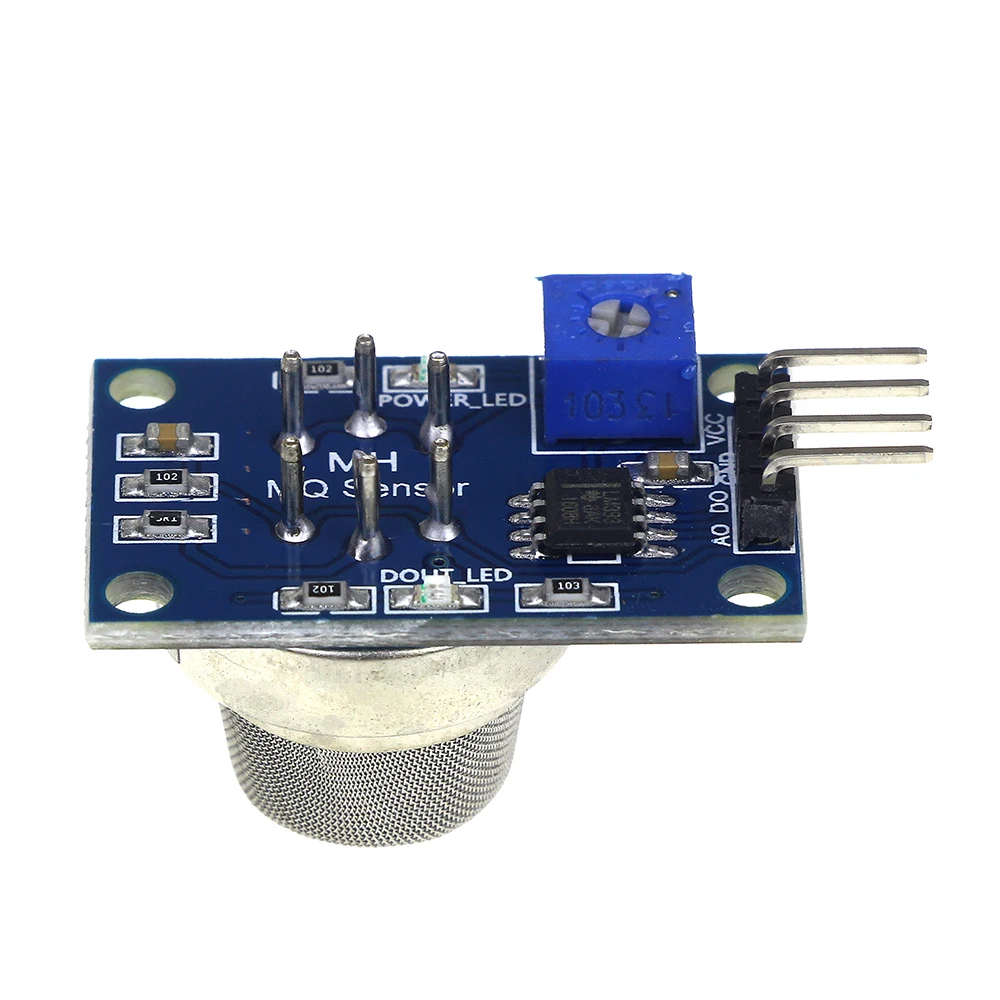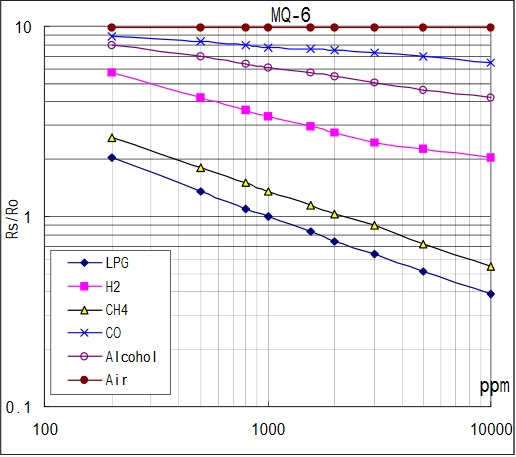# LPG Sensor with MQ-6 and Arduino

The MQ-6 is a semiconductor device for detecting the levels of propane and butane in the air. Since liquefied petroleum gas (LPG) is composed of these two gases, then the MQ-6 can be used as a LPG sensor.

### Introduction

For this tutorial, I will be using the MQ-6 breakout board:Similar to MQ-3 Alcohol sensor, the MQ-6 has four output pins: A0, D0, GND and VCC. A0 gives out varying voltages corresponding to the level of LPG in the air. D0 goes high when a certain threshold is reached. Otherwise, it stays low. The threshold can be varied by adjusting the trimmer on the board.

The datasheet shows the log-log graph of the resistance of the MQ-6 board with 1000 ppm of LPG (R0) to its resistance when other gases are present (RS):While it’s able to read other gases, the MQ-6 is most sensitive to LPG based on the graph. We also see that RS/R0 is equal to a constant 10 in air. This fact is useful when we need to calculate the actual LPG concentration (in PPM) later on.

### MQ-6 Gas Leak Detector

If you want to detect only the presence of LPG in the air, you only need to connect the AO pin to any analog pin of the Arduino UNO. Then you must determine the threshold value by placing the MQ-6 near a safe LPG source (like an unignited gas stove) and record the reading.

Note that the MQ-6 needs at least 20 seconds to warm up.

You can use this Arduino sketch for determining the threshold value. Connect the AO pin to the Arduino UNO’s A0 pin:

For example, if the value in the serial monitor reaches 1.4 V when near the LPG source, then that is your threshold value. You can then proceed to using this sketch:

Of course, 1.4V is just an example. You must test your device to determine the actual threshold value.

### MQ-6 LPG Concentration Sensor

Displaying the LPG concentration in PPM is much more trickier than just specifying a threshold value. Here, the graph presented above is useful. We need to determine two points from the graph to create an equation. Note that the plot is a log-log plot similar to those other MQ gas sensors.

We select the points:

For any line in a log-log plot, the function is as follows:Here, we substitute  F0 = 1, x0 = 1000 and F1 = 0.2, x1 = 10000.

The relationship between RS/R0 and ppm is therefore:

Solving for PPM:

We can determine the LPG concentration in PPM given the RS/R0 ratio.

Determining the RS/R0 ratio can be done if we determine the R0 of the device first. Recall the R0 is the resistance of the device when 1000 ppm of LPG is present in the air. This is about the same as clean air. In fact, only RS would change for different gases. Noting that RS/R0  = 10 in air according to the graph, we use this sketch to determine R0 of the device (make sure there is no LPG source present when using this):

Note that we skipped the effect of the load RL when we calculated RS in the sketch above since it only presents a minor variation to the actual RS.

Once R0 is determined, we can now use the following sketch to determine the concentration of LPG:

If you find this tutorial helpful or have other questions, kindly drop a comment below!

## 3 thoughts on “LPG Sensor with MQ-6 and Arduino”

1.JAY says:

Hello sir, may i know how you determine these 2 values? y = -30000x + 31000

2.Oreo says:

How about the distance between MQ-6 and LPG source?

3.Meg says:

Hello. I used the first two programs only since i only want to detect the presence of LPG. The first program worked fine - although the value kept decreasing (it went below 0.9) so im not sure if that's correct. But i moved on to the second program to detect the presence of LPG. When i verified the code, it displayed an error message - stray '\342'. Im not sure why. Could you please help??

This site uses Akismet to reduce spam. Learn how your comment data is processed.